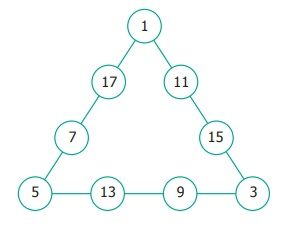Home | | Maths 6th Std | Exercise 6.2

# Exercise 6.2

6th Maths : Term 1 Unit 6 : Information Processing : Exercise 6.2 : Text Book Back Exercises Questions with Answers, Solution

Exercise 6.2

1) In the following magic triangle, arrange the numbers from 1 to 6, so that you get the same sum on all its sides.Solution:2) Using the numbers from 1 to 9(i) Can you form a magic triangle?  Answer: Yes

(ii) How many magic triangles can be formed? Answer: Five

(iii) What are the sums of the sides of the magic triangle? Answer: 17, 19, 20, 21, 23

3) Arrange the odd numbers from 1 to 17 without repetition to get a sum of 30 on each side of the magic triangle.Solution:4) Put the numbers 1, 2, 3, 4, 5, 6, & 7 in the circles so that each straight line of three numbers add up to the same total.Solution:5) Place the number 1 to 12 in the 12 circles so that the sum of the numbers in each of the six lines of the star is 26. Use each number from 1 to 12 exactly once. Find more possible ways?Solution:Exercise 6.2

(1) One of the answers is,(2) (i) Yes (ii) 5 (iii) 17, 19, 20, 21, 23

(3)(4)(5) There are many other possible ways.Tags : Questions with Answers, Solution | Information Processing | Term 1 Chapter 6 | 6th Maths , 6th Maths : Term 1 Unit 6 : Information Processing
Study Material, Lecturing Notes, Assignment, Reference, Wiki description explanation, brief detail
6th Maths : Term 1 Unit 6 : Information Processing : Exercise 6.2 | Questions with Answers, Solution | Information Processing | Term 1 Chapter 6 | 6th Maths